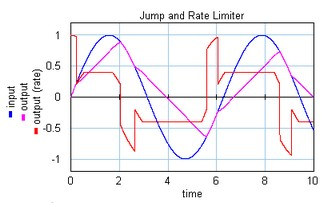﻿ 20-sim webhelp > Library > Signal > Block Diagram Non-Linear > signallimiter-jumpratelimit

# signallimiter-jumpratelimit

## Library

Signal\Block Diagram Non-Linear

## Use

Domains: Discrete, Continuous. Size: 1-D. Allowed in: Block Diagrams.

## Description

This model limits the input signal to a minimum and maximum jump and a minimum and maximum rate.

for minimum < input < maximum:

output = input

else

doutput/dt = dinput/dt; (minimumrate < dinput/dt < maximumrate)

doutput/dt = minimum; (dinput/dt < minimumrate)

output = maximum (dinput/dt > maximumrate)

En example is shown below where a sinusoidal input signal is limited to a minmum jump of -0.2, a maximum jump of 0.2, a maximum rate of 0.4 and a minimum rate of -0.4.## Interface

 Inputs Description input The inputs signal to be limited Outputs output The limited output signal Parameters minimum maximum minimumrate maximumrate Minimum jump of the output. Maximum jump of the output. Minimum rate of the output. Maximum rate of the output.